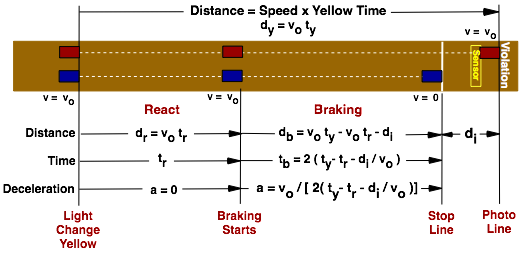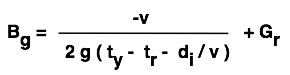# Models and Proof

Deceleration Model | Driver Braking (g's) | Yellow Light Duration Formula
Deceleration Model
The braking (deceleration) model determines the point a vehicle must stop based on speed, reaction time, yellow duration, and distance between the stop line and camera trigger. The model also determines the braking (deceleration) for a vehicle whose driver decides to stop.
Deceleration ModelProof GIVEN Vo = Speed tr = driver reaction time ty = yellow light duration di = distance between stop line and photo line -- SOLVE FOR dr = distance traveled during react time db = distance traveled during braking time tb = braking time -a = deceleration

 Four unknowns (dr, db, tb, a) and 4 equations. From Layout db = dy - dr - di db = Voty - Votr - di db = Vo(ty - tr) - di | -----> From Newton's Law of Motion db = Votb + 0.5 a tb2 db = Vo tb + 0.5 (-Vo / tb) tb2 db = Vo tb - 0.5 Vo tb db = 0.5 Vo tb tb = 2 db / Vo From Layout a = -Vo / tb <----- By Definition dr = Vo tr dy = Vo ty tb = 2 (Vo ty - Vo tr - di) / Vo tb = 2 (ty - tr - di/Vo) a = - Vo/tb = -Vo / [ 2 (ty - tr - di/Vo) ]

Solving for Yellow Duration (ty)
ty = tr - (Vo/2a) + di/Vo
Applies to flat level surfaces, see below for inclines.
Note, (a) is negative for deceleration (-a).
INCLINES
Inclines (because of gravity) effect braking by making it easier to brake uphill, and harder downhill. The difference is a function of gravity and the ratio of elevation change to horizontal distance (road grade ratio Gr). Gr is positive for uphill (+Gr), negative for downhill (-Gr), and zero for level (Gr = 0).
a = af + g Gr
af = Acceleration (-af deceleration) on a flat surface
g = acceleration due to gravity constant (see below)

Accounting for inclines Acceleration becomes;
a = -vo / [ 2 (ty - tr - di/vo) ] + g Gr

Driver Braking (g's)
Deceleration can be equated to the Gravitational Constant (g), acceleration due to gravity. By International definition 1 g is a change in speed (acceleration, sometimes mistakenly confused with force) of exactly 9.80665 meters per second every second (32.17405 ft/s2).
Braking g's = Bg = a / gBg = Braking g's v = Speed tr = Driver Reaction Time ty = Yellow Light Duration di = Distance Between Stop Line and Photo Line Gr = Road Grade Ratio, +Gr uphill, -Gr downhill g = Gravitational Constant = 9.80665 m/s2 = 32.17405 ft/s2

## Yellow Light Duration Formula

Yellow Light Time (ty)
ty = tr + { V / [ 2 ( a + g Gr ) ] } + di / V

 ty = tr + V---------------- + di----- 2 (a + g Gr) V

 ty = Yellow Light Duration tr = driver Reaction Time V = initial Speed a = Deceleration di = Distance from Stop Line to Photo Line Gr = road Grade ratio, +Gr uphill, -Gr downhill g = Gravitational Constant